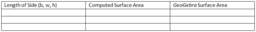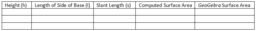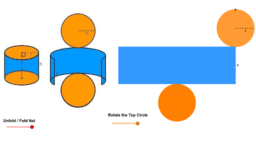# CCSS Gr. 6 Nets and Surface Area

Topic:
Geometry
Common Core Standards for Grade 6-Geometry Solve real-world and mathematical problems involving area, surface area, and volume. 6.G.1. Find the area of right triangles, other triangles, special quadrilaterals, and polygons by composing into rectangles or decomposing into triangles and other shapes; apply these techniques in the context of solving real-world and mathematical problems. 6.G.2. Find the volume of a right rectangular prism with fractional edge lengths by packing it with unit cubes of the appropriate unit fraction edge lengths, and show that the volume is the same as would be found by multiplying the edge lengths of the prism. Apply the formulas V = l w h and V = b h to find volumes of right rectangular prisms with fractional edge lengths in the context of solving real-world and mathematical problems. 6.G.3. Draw polygons in the coordinate plane given coordinates for the vertices; use coordinates to find the length of a side joining points with the same first coordinate or the same second coordinate. Apply these techniques in the context of solving real-world and mathematical problems. 6.G.4. Represent three-dimensional figures using nets made up of rectangles and triangles, and use the nets to find the surface area of these figures. Apply these techniques in the context of solving real-world and mathematical problems. Apply and extend previous understandings of numbers to the system of rational numbers. Extend coordinate axes familiar from previous grades to represent points on the plane with negative number coordinates.
• Understand signs of numbers in ordered pairs as indicating locations in quadrants of the coordinate plane; recognize that when two ordered pairs differ only by signs, the locations of the points are related by reflections across one or both axes.
• Find and position pairs of integers and other rational numbers on a coordinate plane.
•### FINDING THE SURFACE AREA OF 3-D SHAPES:  THE CUBE

•### FINDING THE SURFACE AREA OF 3-D SHAPES:  THE PYRAMID

•